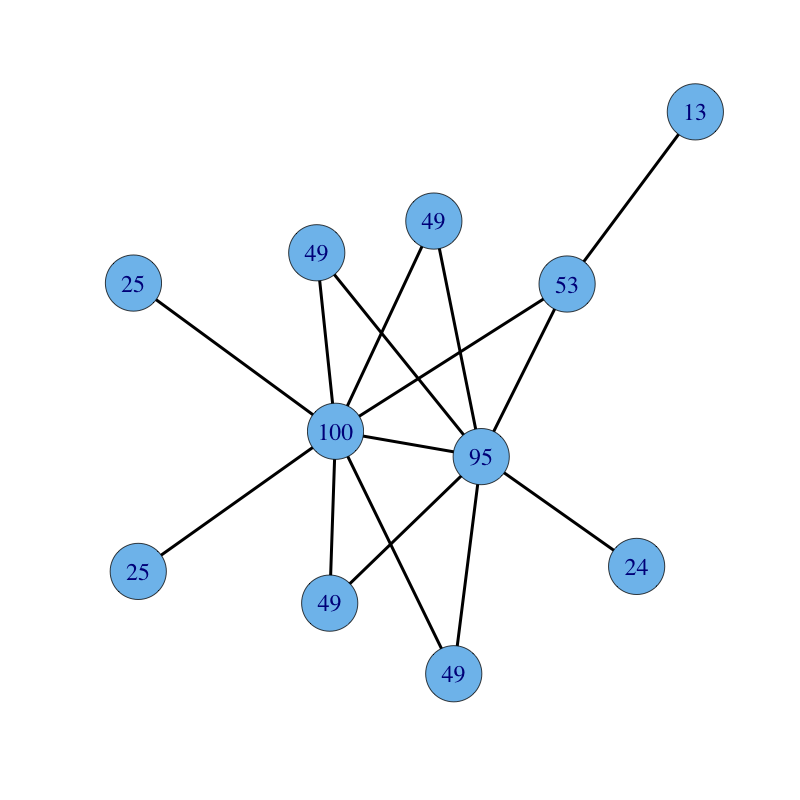## Eigenvector Centrality

A natural extension of degree centrality is eigenvector centrality. In-degree centrality awards one centrality point for every link a node receives. But not all vertices are equivalent: some are more relevant than others, and, reasonably, endorsements from important nodes count more. The eigenvector centrality thesis reads:

A node is important if it is linked to by other important nodes.

Eigenvector centrality differs from in-degree centrality: a node receiving many links does not necessarily have a high eigenvector centrality (it might be that all linkers have low or null eigenvector centrality). Moreover, a node with high eigenvector centrality is not necessarily highly linked (the node might have few but important linkers).

Eigenvector centrality, regarded as a ranking measure, is a remarkably old method. Early pioneers of this technique are Wassily W. Leontief (The Structure of American Economy, 1919-1929. Harvard University Press, 1941) and John R. Seeley (The net of reciprocal influence: A problem in treating sociometric data. The Canadian Journal of Psychology, 1949).

### Math

Let $A = (a_{i,j})$ be the adjacency matrix of a graph. The eigenvector centrality $x_{i}$ of node $i$ is given by: $$x_i = \frac{1}{\lambda} \sum_k a_{k,i} \, x_k$$ where $\lambda \neq 0$ is a constant. In matrix form we have: $$\lambda x = x A$$

Hence the centrality vector $x$ is the left-hand eigenvector of the adjacency matrix $A$ associated with the eigenvalue $\lambda$. It is wise to choose $\lambda$ as the largest eigenvalue in absolute value of matrix $A$. By virtue of Perron-Frobenius theorem, this choice guarantees the following desirable property: if matrix $A$ is irreducible, or equivalently if the graph is (strongly) connected, then the eigenvector solution $x$ is both unique and positive.

The power method can be used to solve the eigenvector centrality problem. Let $m(v)$ denote the signed component of maximal magnitude of vector $v$. If there is more than one maximal component, let $m(v)$ be the first one. For instance, $m(-3,3,2) = -3$. Let $x^{(0)}$ be an arbitrary vector. For $k \geq 1$:

1. repeatedly compute $x^{(k)} = x^{(k-1)} A$;
2. normalize $x^{(k)} = x^{(k)} / m(x^{(k)})$;

until the desired precision is achieved. It follows that $x^{(k)}$ converges to the dominant eigenvector of $A$ and $m(x^{(k)})$ converges to the dominant eigenvalue of $A$. If matrix $A$ is sparse, each vector-matrix product can be performed in linear time in the size of the graph.

The method converges when the dominant (largest) and the sub-dominant (second largest) eigenvalues of $A$, respectively denoted by $\lambda_1$ and $\lambda_2$, are separated, that is they are different in absolute value, hence when $|\lambda_1| > |\lambda_2|$. The rate of convergence is the rate at which $(\lambda_2 / \lambda_1)^k$ goes to $0$. Hence, if the sub-dominant eigenvalue is small compared to the dominant one, then the method quickly converges.

### Code

The built-in function evcent (R, C) computes eigenvector centrality.

A user-defined function eigenvector.centrality follows:

 # Eigenvector centrality (direct method) #INPUT # g = graph # t = precision # OUTPUT # A list with: # vector = centrality vector # value = eigenvalue # iter = number of iterations eigenvector.centrality = function(g, t) { A = get.adjacency(g); n = vcount(g); x0 = rep(0, n); x1 = rep(1/n, n); eps = 1/10^t; iter = 0; while (sum(abs(x0 - x1)) > eps) { x0 = x1; x1 = as.vector(x1 %*% A); m = x1[which.max(abs(x1))]; x1 = x1 / m; iter = iter + 1; } return(list(vector = x1, value = m, iter = iter)) } 

### Example

An undirected connected graph with nodes labelled with their eigenvector centrality follows: